#代码审计  #codeql

# 2.CodeQL语法#

## 前言#

CodeQL的很多语法和现在的主流高级语言有很多相似之处，但也有许多的不同，学习的时候需要注意。

## 基础数据类型(Primitive types)#

CodeQL 是一种静态类型的语言，因此每个变量都必须有一个声明的类型。类型是一组值。例如，int 类型是一组整数。注意，一个值可以属于这些集合中的多个，这意味着它可以有多个类型。

### 日期型(date)#

``````from date start, date end
where start = "01/09/2021".toDate() and end = "02/11/2021".toDate()
select start.daysTo(end)
``````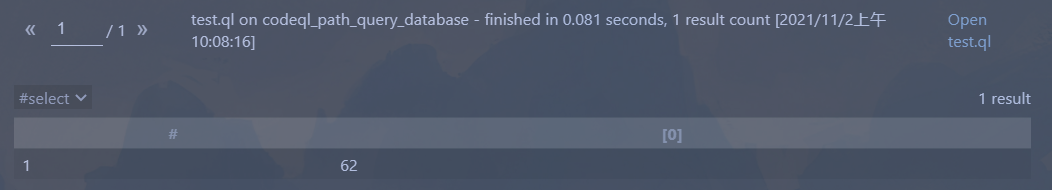- string - go
- date - go

### 布尔型(boolean)#

``````from boolean a, boolean b
where a = true and b = false
select a.booleanAnd(b)
``````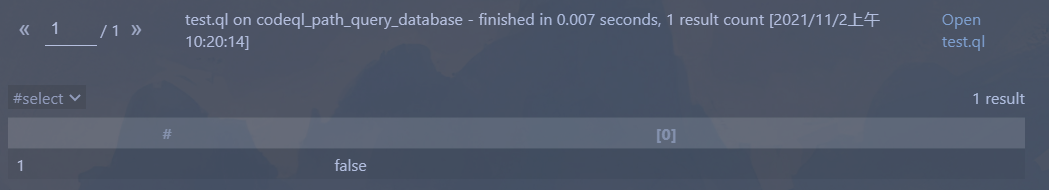- boolean - go

## 谓词(Predicates)#

1. 需要注意的是谓词的名字开头必须是小写字母。
2. 绑定行为与绑定集，这个在后面会介绍。

### 无结果谓词#

``````predicate isCity(string city) {
city = "Beijing"
or
city = "ShangHai"
}

from string city
where city = "Beijing" and isCity(city)
select city
``````

### 结果谓词#

``````int addOne(int i) {
result = i + 1 and
i in [1 .. 10]
}

from int v
where v = 1
``````

### 递归谓词#

``````string getANeighbor(string country) {
country = "France" and result = "Belgium"
or
country = "France" and result = "Germany"
or
country = "Germany" and result = "Austria"
or
country = "Germany" and result = "Belgium"
or
country = getANeighbor(result)
}

from string people
where people = getANeighbor("Germany")
select people
``````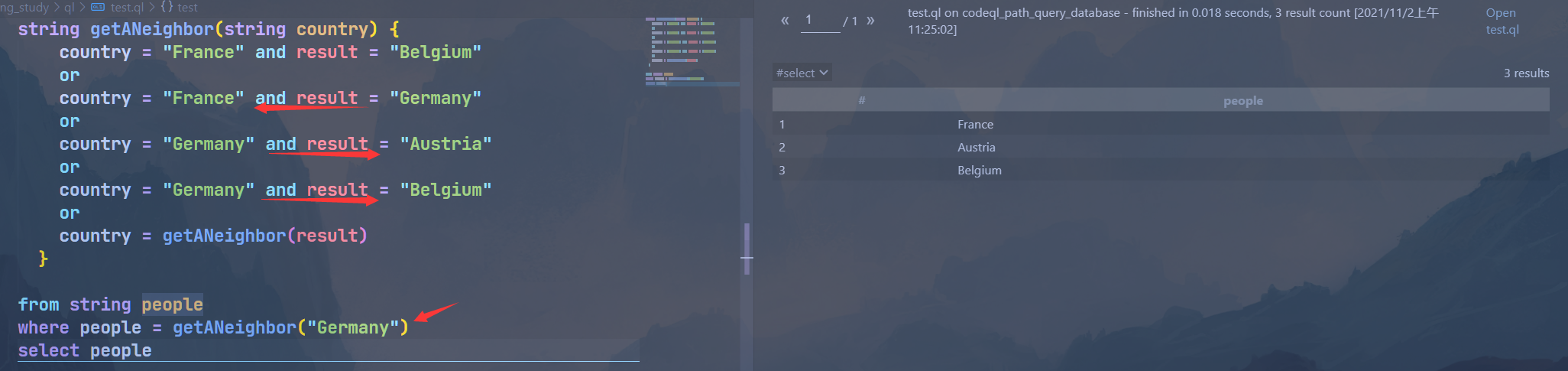### 特征谓词，非成员谓词，成员谓词#

``````int getSuccessor(int i) {  // 1. Non-member predicate
result = i + 1 and
i in [1 .. 9]
}

class FavoriteNumbers extends int {
FavoriteNumbers() {  // 2. Characteristic predicate
this = 1 or
this = 4 or
this = 9
}

string getName() {   // 3. Member predicate for the class `FavoriteNumbers`
this = 1 and result = "one"
or
this = 4 and result = "four"
or
this = 9 and result = "nine"
}
}
``````

### 绑定行为与绑定集#

``````// 正例，i被限定在1到10内，或者你也可以给i赋一个确定的值如i=1
result = i + 1 and
i in [1 .. 10]
}
``````

``````// 反例，i是无限数量值的，此时CodeQL编译器会报错: 'i' is not bound to a value
result = i + 1 and
i > 0
}
``````

#### 单个绑定集#

``````bindingset[i]
result = i + 1 and
i > 0
}

// 此时我们可以去调用这个谓词，但是需要注意传递过来的参数还是只能在有限的参数集中
from int i
where i = 1
``````

#### 多个绑定集#

``````bindingset[x] bindingset[y]
predicate plusOne(int x, int y) {
x + 1 = y
}
``````

``````bindingset[x, y]
predicate plusOne(int x, int y) {
x + 1 = y
}
``````

## 查询(Query)#

- select子句
- 查询谓词，这意味着我们可以在当前模块中定义或者从其他模块中导入

### select子句#

select子句的格式如下:

``````[from] /* ... variable declarations ... */
[where] /* ... logical formula ... */
select /* ... expressions ... */
``````

- `as`关键字，后面跟随一个名字。作用相当于sql中的`as`，为结果列提供了一个"标签"，并允许在后续的select表达式中使用它们。
- `order by`关键字，后面跟随一个一个结果列名。作用相当于sql中的`order by`，用于排序结果，并且在结果列名后可选`asc`(升序)或`desc`(降序)关键字。

``````from int x, int y
where x = 3 and y in [0 .. 2]
select x, y, x * y as product, "product: " + product
``````

### 查询谓词#

``````query int getProduct(int x, int y) {
x = 3 and
y in [0 .. 2] and
result = x * y
}
``````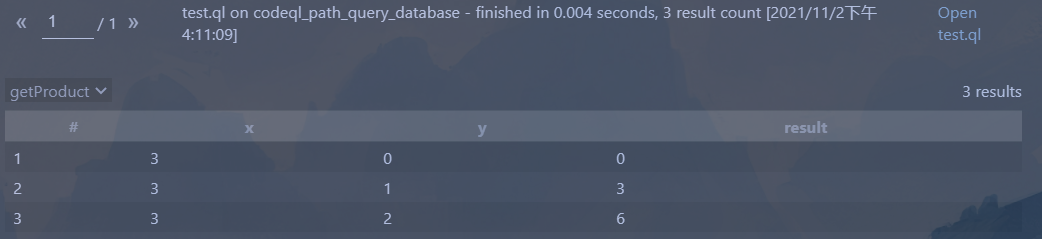``````query int getProduct(int x, int y) {
x = 3 and
y in [0 .. 2] and
result = x * y
}
class MultipleOfThree extends int {
MultipleOfThree() { this = getProduct(_, _) }
}

from MultipleOfThree m
select m
``````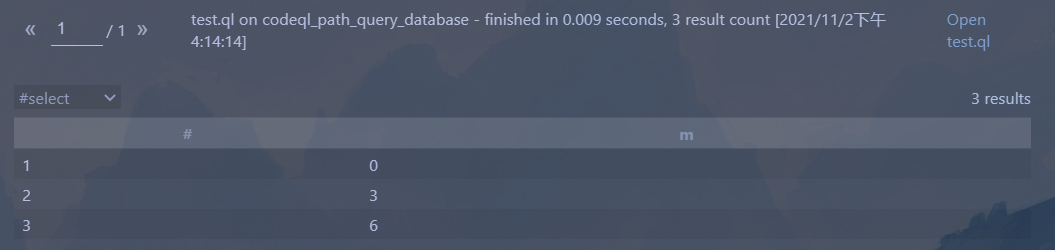## 类(Classes)#

- 在类中定义成员谓词
- 定义子类以重写成员谓词

### 类的定义#

``````class ClassName [extends Parent] {
// ...
}
``````

``````class OneTwoThree extends int {
OneTwoThree() { // characteristic predicate
this = 1 or this = 2 or this = 3
}

string getAString() { // member predicate
result = "One, two or three: " + this.toString()
}

predicate isEven() { // member predicate
this = 2
}
}
``````

- 不能继承本身
- 不能继承final类
- 不能继承不兼容的类型，请参阅类型兼容性

### 类的主体#

- 一个特征谓词
- 任意数量的成员谓词
- 任意数量的字段(field)

#### 成员谓词#

``````(OneTwoThree).getAString()
// 结果是 One, two or three: 1
``````

#### 字段(Field)#

``````class SmallInt extends int {
SmallInt() { this = [1 .. 10] }
}

class DivisibleInt extends SmallInt {
SmallInt divisor;   // declaration of the field `divisor`
DivisibleInt() { this % divisor = 0 }

SmallInt getADivisor() { result = divisor }
}

from DivisibleInt i
``````

### 抽象类#

``````import go

abstract class SqlExpr extends Expr {
int ID;
}

class PostgresSqlExpr extends SqlExpr {
PostgresSqlExpr(){ ID=1 }
}

class MySqlExpr extends SqlExpr {
MySqlExpr(){ ID=2 }
}
``````

### 重写成员谓词#

``````class OneTwo extends OneTwoThree {
OneTwo() {
this = 1 or this = 2
}

override string getAString() {
result = "One or two: " + this.toString()
}
}
``````

``````from OneTwoThree o
select o, o.getAString()
``````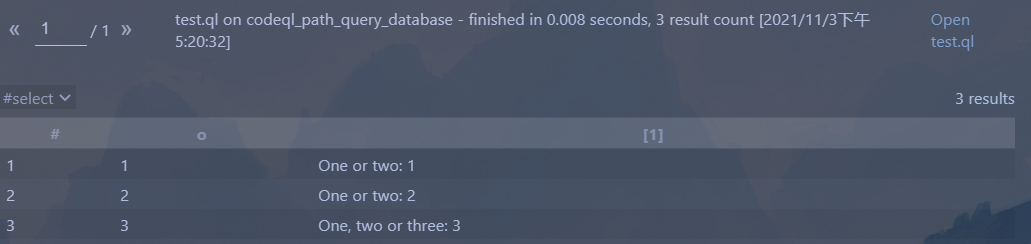``````class TwoThree extends OneTwoThree {
TwoThree() {
this = 2 or this = 3
}

override string getAString() {
result = "Two or three: " + this.toString()
}
}
``````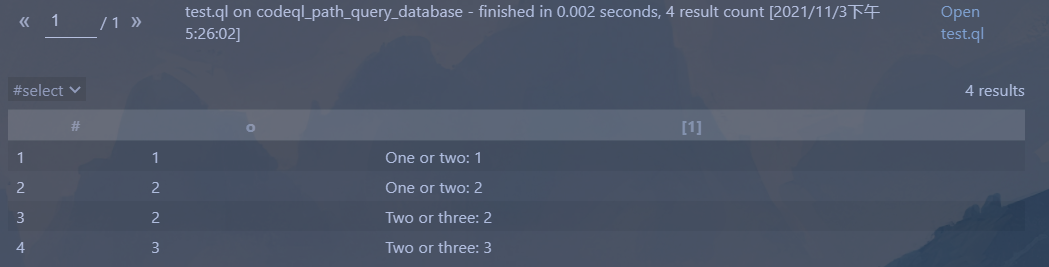### 多重继承#

``````class Two extends OneTwo, TwoThree {}
``````

``````class Two extends OneTwo, TwoThree {
override string getAString() {
result = TwoThree.super.getAString()
}
}
``````

### 非继承子类(Non-extending subtypes)

## 模块(Modules)

### 模块主体

- 导入模块语句
- 谓词
- 类型
- 别名
- 显式模块
- select子句(仅可在查询模块中使用)

### 定义模块

`````ql module Example { class OneTwoThree extends int { OneTwoThree() { this = 1 or this = 2 or this = 3 } } } ```

``````### 导入模块

```ql
import <module_expression1> as <name>
import <module_expression2>
``````
``` 这相当于python中的```from import *`

### 模块种类#

#### 查询模块#

- 无法导入其他查询模块
- 一个查询模块必须包含一个查询，它可以是select语句或者一个查询谓词

#### 显式模块#

``````...
module M {
class OneTwo extends OneTwoThree {
OneTwo() {
this = 1 or this = 2
}
}
}
``````